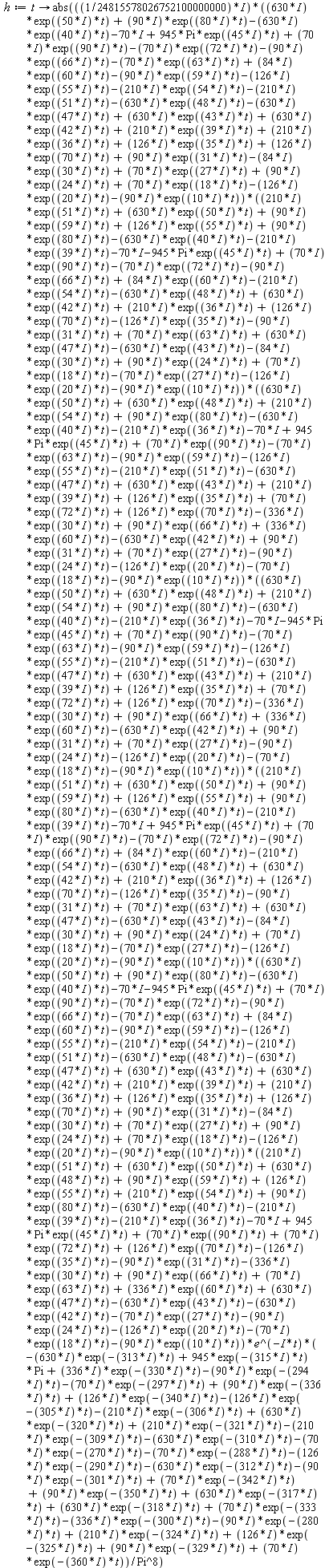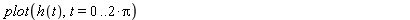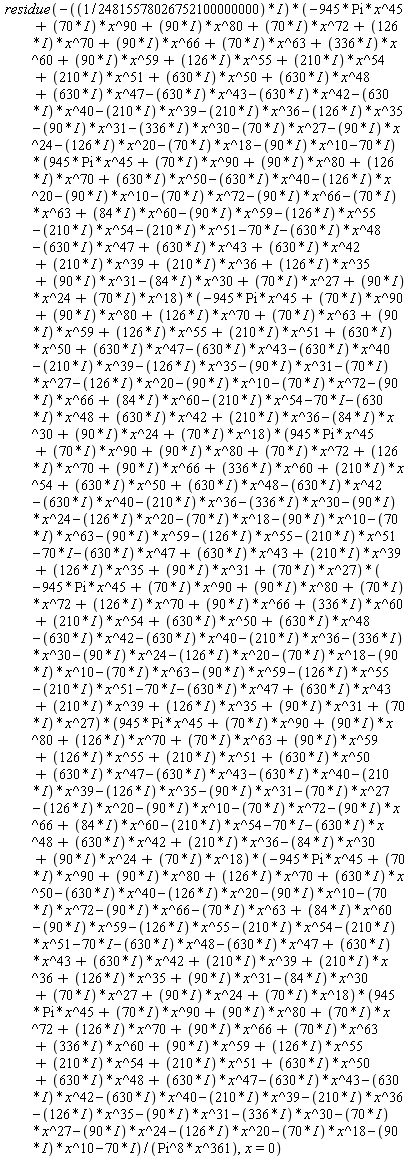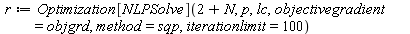## 99 Reputation

15 years, 242 days

## how to remove duplicates from a set or l...

Maple 2015

The set and list produced by map (see below) contain duplicates.  How to remove duplicates?

 >(1)
 >(2)
 >(3)
 >(4)
 >(5)
 >(6)
 >(7)
 >(8)
 >(9)
 >(10)
 >(11)
 >(12)
 >## How do I get a meaningful error message?...

Maple

I get the following message:

"Error, (in Bits:-GetBits) argument 1, the number, must be a nonnegative integer"

Presumably "argument 1" does not mean that the offending argument is 1 (the problem is that the offending argument "must be a nonnegative integer", but 1 is a nonnegative integer).  So how do I get maple to report what the offending argument is?

## how to get nonempty plot?...

Maple 2015I would like to plot h over the real interval from 0 to 2*Pi, butproduces an empty plot...  How can I plot successfully?

## how to make residue command work?...

Maple 2015

If I enter the following I do not get the residue... how do I make the residue command work?## How to export result from Optimization[N...

I can't cut and paste from my maple worksheet (because syntax somehow gets changed in the process), so perhaps the following attempt at carefully worded  prose will do (alternately, please someone tell me how to attach screen shots).  Here goes...

I executeThe optimization succeeds and the assignment takes place.  The result is what I'ld like to cut and paste so youall can see...  it looks as follows. 'r:=' (but no quotes)  followed by open square bracket, then a number, then a comma, then another open square bracket, then a vertically arranged list of stuff, then two close brackets.  I presume the vertically arranged stuff in the innermost matching square brackets somehow represents the point in the search space where the minimum takes place, and the minimum is the number that follows the first square bracket and is before the comma.  My goal is to write to disk the the point in the search space where the minimum takes place (I suppose that vector is in some sense r) .  My attempt to write it to disk is

ExportMatrix("maple.txt", r, target = auto)

but maple complains

ExportMatrix("maple.txt", r, target = auto);
Error, invalid input: ExportMatrix expects its 2nd argument, M, to be of type {Matrix, list(Matrix)}, but received [293148935266.099426, Vector(28, {(1) = -233674.34818878854, (2) = 12.62636610207248, (3) = 0.3337043409389546e-2, (4) = 0.13303915611938133e-1, (5) = .0, (6) = .2589485687872699, (7) = 0.9341600279762804e-1, (8) = 0.3293862590239189e-1, (9) = 0.12892649003200331e-1, (10) = 0.606528143324688e-1, (11) = 0.17524827222051658e-1, (12) = -0.16059611469561555e-17, (13) = 0.621465506367271e-1, (14) = .29826235341855, (15) = -0.16288776533795546e-17, (16) = 0.15301368661023716e-1, (17) = 0.1529933711903137e-18, (18) = 0.4375115591744606...

How can I write the result of optimization to disk as a plain ascii text file (tsv format)?

Thanks

 1 2 3Page 1 of 3
﻿